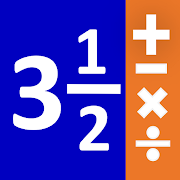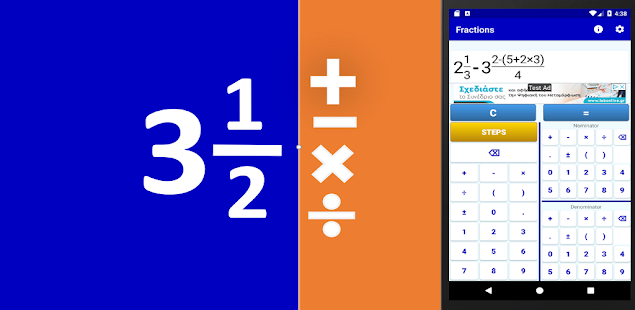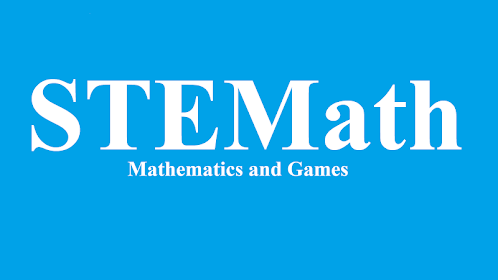# Fractions - Fraction Calculator for school mathsEveryone
934
·
Offers in-app purchasesThis app is a simple fraction calculator that performs analytic calculations between math fractions. It has an elegant input system and gives analytic step by step solutions.
It is perfect for students and teachers that work with fractions and maths!

The fraction calculator supports:
1) All fraction operations (fraction multiplication, fraction division, fraction addition, fraction subtraction)
2) Fraction simplification
3) Mixed Numbers, Integer Numbers and Fractions
4) Step by step solution for all fraction computations
5) Any number of parentheses
6) The calculator shows the result as a fraction, or as a mixed number and it shows a decimal approximation.
7) Fraction to decimal calculator/converter
8) Decimal to fraction calculator/converter

You can use the fraction calculator either for directly evaluating a math expression with fractions and using the final result, or for studying the steps of calculations for math classes.

-----------------------------------------------------
A. How to input a fraction?
The calculator has three number fields. The leftmost one is used to enter the integer part of the fraction or to enter an operator (+, -, *, :), or a parenthesis. On the right side there are two more number fields. The one in the top right is used to enter the fraction's numerator, while the one in the bottom right is used to enter the fraction's denominator.
Thus if you want to enter the mixed fraction 5 1/2, you should press the number 5 on the left number field, then the number 1 on the right top number field and finally the number 2 on the right bottom number field.

B. How to compute an expression with fractions?
Just enter the required expression and then press the equals ("=") button of the calculator.

C. How to simplify a fraction?
Just enter the fraction and press the equals ("=") button of the calculator.

D. How to convert a mixed fraction to a simple fraction?
Just enter the mixed fraction and press the equals ("=") button of the calculator.

D. How to convert an improper fraction to a mixed fraction?
You should change the way the calculator shows the result of the computations. Press the gear (ont he top right of the application bar) and select how you want the app to show the result on the screen. If you choose "Mixed Fractions" then each expression you enter will be converted to a mixed fraction. For example if you enter the fraction 11/2 and then press the equals ("=") button, the calculator will show the mixed fraction 5 1/2 on the screen. You can go back to the original setting using the gear button and selecting "Simple Fractions".

E. How to convert a decimal number to a fraction.
Just enter the decimal number and press the equals ("=") button of the calculator.

F. How to convert a fraction to a decimal number.
Just enter the decimal number and press the equals ("=") button of the calculator. On top of the result the application shows the decimal approximation of the resulting fraction.
Collapse

## Reviews

Review policy and info
4.5
934 total
5
4
3
2
1

Updated
September 20, 2021
Size
2.8M
Installs
100,000+
Current Version
1.6
Requires Android
7.0 and up
Content Rating
Everyone
In-app Products
\$1.49 per item
Permissions
Offered By
STEMath
Developer
Spirou Lambrou 88, Pireas, 18539, Greece.